### Practice Set 1.4 Similarity Class 10th Mathematics Part 2 MHB Solution

Practice Set 1.4
1. The ratio of corresponding sides of similar triangles is 3 : 5; then find the ratio of…
2. If ΔABC ~ ΔPQR and AB: PQ = 2:3, then fill in the blanks. a (deltaabc)/a (deltapqr) =…
3. If ΔABC ~ ΔPQR, A (ΔABC) = 80, A(ΔPQR) = 125, then fill in the blanks. a (deltaabc)/a…
4. ΔLMN ~ ΔPQR, 9 × A(ΔPQR) = 16 × A (ΔLMN). If QR = 20 then find MN.…
5. Areas of two similar triangles are 225 sq.cm 81 sq.cm. If a side of the smaller…
6. Δ ABC and ΔDEF are equilateral triangles. If A(ΔABC) : A(ΔDEF) = 1 : 2 and AB = 4, find…
7. In figure 1.66, seg PQ || seg DE, A(Δ PQF) = 20 units, PF = 2 DP, then find A(DPQE) by…
###### Practice Set 1.4
Question 1.

The ratio of corresponding sides of similar triangles is 3 : 5; then find the ratio of their areas.

Theorem: When two triangles are similar, the ratio of areas of those triangles is equal to the ratio of the squares of their corresponding sides.

⇒ Ratio of areas = 32:52

⇒ Ratio of areas = 9 : 25

Question 2.

If ΔABC ~ ΔPQR and AB: PQ = 2:3, then fill in the blanks.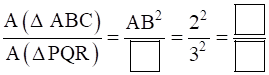∵ Δ ABC~Δ PQR and AB:PQ = 2:3

⇒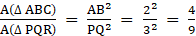Question 3.

If ΔABC ~ ΔPQR, A (ΔABC) = 80, A(ΔPQR) = 125, then fill in the blanks.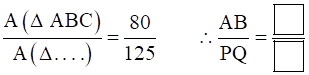∵ Δ ABC~Δ PQR

⇒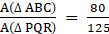(∵ A(Δ PQR) = 125 is given)

⇒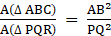⇒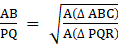⇒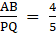Question 4.

ΔLMN ~ ΔPQR, 9 × A(ΔPQR) = 16 × A (ΔLMN). If QR = 20 then find MN.

∵ Δ ABC~Δ PQR

⇒ Given that, 9× A(Δ ABC) = 16× A(Δ PQR)

⇒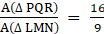And,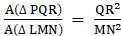⇒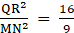⇒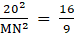⇒ MN2 =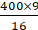⇒ MN = 15

Question 5.

Areas of two similar triangles are 225 sq.cm & 81 sq.cm. If a side of the smaller triangle is 12 cm, then find corresponding side of the bigger triangle.

Let area of one(bigger) triangle be ‘A’, other(smaller) triangle be ‘B’,corresponding side of smaller triangle be ‘a’ and bigger triangle be ‘b’.

⇒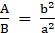(By theorem)

And a = 12cm, A = 225 sq.cm, B = 81 sq.cm ……(Given)

⇒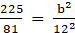⇒ b2 =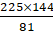⇒ b = √400

⇒ b = 20 cm

Question 6.

Δ ABC and ΔDEF are equilateral triangles. If A(ΔABC) : A(ΔDEF) = 1 : 2 and AB = 4, find DE.

We know that, all the angles of an equilateral triangles are equal, i.e., 60°.

⇒ Δ ABC~Δ DEF ……(By AAA Similarity Test)

⇒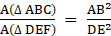And,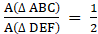(Given)

⇒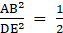⇒ DE2 = 2× 42 (∵ AB = 4)

⇒ DE = √32

DE = 4√2

Question 7.

In figure 1.66, seg PQ || seg DE, A(Δ PQF) = 20 units, PF = 2 DP, then find A(DPQE) by completing the following activity.

A(Δ PQF) = 20 units, PF = 2 DP, Let us assume DP = x. ∴ PF = 2x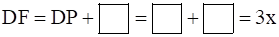In Δ FDE and Δ FPQ,

∠FDE ≅ ∠ .......... corresponding angles

∠FED ≅ ∠ ......... corresponding angles

∴ Δ FDE ~ Δ FPQ .......... AA test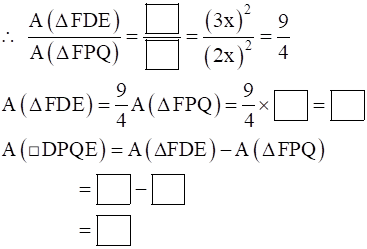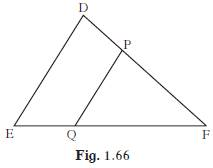A(Δ PQF) = 20units,PF = 2DP,Let us assume DP = x,∴ PF = 2x

⇒ DF = DP + PF = x + 2x = 3x

In Δ FDE & Δ FPQ

∠ FDE ≅ ∠ FPQ (Corresponding angles)

∠ FEP ≅ ∠ FQP (Corresponding angles)

∴ Δ FDE~ Δ FPQ (AA Test)

∴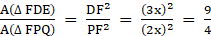A(Δ FDE) =A(ΔFPQ) =× 20 = 45

A(□DPQE) = A (Δ FDE) - A(ΔFPQ)

= 45-20

= 25 sq.unit.

## PDF FILE TO YOUR EMAIL IMMEDIATELY PURCHASE NOTES & PAPER SOLUTION. @ Rs. 50/- each (GST extra)

SUBJECTS

HINDI ENTIRE PAPER SOLUTION

MARATHI PAPER SOLUTION
SSC MATHS I PAPER SOLUTION
SSC MATHS II PAPER SOLUTION
SSC SCIENCE I PAPER SOLUTION
SSC SCIENCE II PAPER SOLUTION
SSC ENGLISH PAPER SOLUTION
SSC & HSC ENGLISH WRITING SKILL
HSC ACCOUNTS NOTES
HSC OCM NOTES
HSC ECONOMICS NOTES
HSC SECRETARIAL PRACTICE NOTES

2019 Board Paper Solution

HSC ENGLISH SET A 2019 21st February, 2019

HSC ENGLISH SET B 2019 21st February, 2019

HSC ENGLISH SET C 2019 21st February, 2019

HSC ENGLISH SET D 2019 21st February, 2019

SECRETARIAL PRACTICE (S.P) 2019 25th February, 2019

HSC XII PHYSICS 2019 25th February, 2019

CHEMISTRY XII HSC SOLUTION 27th, February, 2019

OCM PAPER SOLUTION 2019 27th, February, 2019

HSC MATHS PAPER SOLUTION COMMERCE, 2nd March, 2019

HSC MATHS PAPER SOLUTION SCIENCE 2nd, March, 2019

SSC ENGLISH STD 10 5TH MARCH, 2019.

HSC XII ACCOUNTS 2019 6th March, 2019

HSC XII BIOLOGY 2019 6TH March, 2019

HSC XII ECONOMICS 9Th March 2019

SSC Maths I March 2019 Solution 10th Standard11th, March, 2019

SSC MATHS II MARCH 2019 SOLUTION 10TH STD.13th March, 2019

SSC SCIENCE I MARCH 2019 SOLUTION 10TH STD. 15th March, 2019.

SSC SCIENCE II MARCH 2019 SOLUTION 10TH STD. 18th March, 2019.

SSC SOCIAL SCIENCE I MARCH 2019 SOLUTION20th March, 2019

SSC SOCIAL SCIENCE II MARCH 2019 SOLUTION, 22nd March, 2019

XII CBSE - BOARD - MARCH - 2019 ENGLISH - QP + SOLUTIONS, 2nd March, 2019

HSC Maharashtra Board Papers 2020

(Std 12th English Medium)

HSC ECONOMICS MARCH 2020

HSC OCM MARCH 2020

HSC ACCOUNTS MARCH 2020

HSC S.P. MARCH 2020

HSC ENGLISH MARCH 2020

HSC HINDI MARCH 2020

HSC MARATHI MARCH 2020

HSC MATHS MARCH 2020

SSC Maharashtra Board Papers 2020

(Std 10th English Medium)

English MARCH 2020

HindI MARCH 2020

Hindi (Composite) MARCH 2020

Marathi MARCH 2020

Mathematics (Paper 1) MARCH 2020

Mathematics (Paper 2) MARCH 2020

Sanskrit MARCH 2020

Important-formula

THANKS